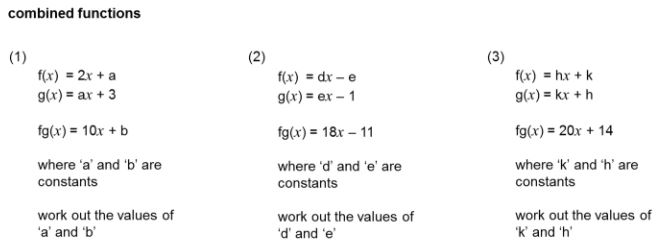# FunctionsA previous post on Functions features resources aimed at older students; I thought it would be useful to look at resources for teaching younger students.

For an excellent introduction the PhET Simulation, Function Builder provides a very visually appealing resource. Using the pattern option even very young students could explore important concepts. This is very intuitive to use, the slides in the following slideshow demonstrate examples of its use. Note that teachers can register with PhET and gain access to supporting documentation.

Desmos can be used very simply to illustrate function notation and note the use of Desmos as a calculator to evaluate the value of a function for a given input.

As always CIMT is worth a search; we find:

In the interactive materials for year 7: Unit 16 Section 2: Function Machines and there is also a section in the associated text (16.2). All CIMT resources are free to access, a small number of documents such as text answers are password protected, you can obtain the CIMT password. These exercises could be used as an introduction and students also shown function notation. They could write the functions described by the function machines using f(x) notation.

On Transum, try this online exercise on function notation, inverse functions and composite functions.

On Maths Genie, under Grade 7, we have Inverse and Composite Functions. You will find some revision examples and also exam questions with solutions.

From Don Steward, try these lovely questions on Compound Functions.On TES, this Crossnumber from cbarthur is an ideal resource for becoming familiar with function notation. Also on TES from Owen134866 we have a set PowerPoint resources including an introduction to f(x) notation for GCSE students.

The AQA Bridging the Gap resources includes a resource on introducing function notation; these resources are ideal for students who have completed the previous KS3 programmes of study but will be studying the new GCSE courses.

From OCR, it strikes me that the Check in tests will be very useful in KS3, consider the language of functions for example, the first questions could be used for students as young as Year 7 (age 11-12).

.

On Exam Solutions you will find an introduction to f(x) notation.